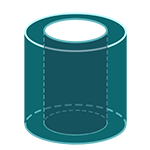Report a Problem
Suggestions

#Calculate The Surface Area of A Hollow Right Cylinder

Last updated: Saturday, April 29, 2023
Select a cylindrical shape below
Right Cylinder
Hollow Cylinder
Oblique Cylinder
Right Truncated
Cylindrical Wedge

The surface area of a hollow right cylinder, also known as a cylindrical shell, is the sum of the surface areas of its two circular faces and its lateral surface area. The lateral surface area is the difference between the surface area of the outer cylinder and the inner cylinder.

To calculate the surface area of a hollow right cylinder, you need to know the outer radius (r), inner radius (R), and the height (h) of the cylinder.

The surface area can be calculated using the formula:

surface area = 2πh(R + r) + 2π(R^2 - r^2)

Real-life examples of cylindrical shells include metal pipes, cans, and drums, which are often used for transportation and storage of liquids and gases. The calculation of their surface area is important for determining the amount of material needed for their production, as well as for their capacity and structural stability.

The formula for determining the surface area of a hollow right cylinder is defined as:
$$SA$$ $$=$$ $$2$$ $$\cdot$$ $$\pi$$ $$\cdot$$ $$h$$ $$\cdot$$ $$(R$$ $$+$$ $$r)$$ $$+$$ $$2$$ $$\cdot$$ $$\pi$$ $$\cdot$$ $$(R^2$$ $$-$$ $$r^2)$$
$$SA$$: the surface area of the hollow cylinder
$$r$$: the radius of the inner ring
$$R$$: the radius of the outer ring
$$h$$: the height of the cylinder
The SI unit of surface area is: $$square \text{ } meter\text{ }(m^2)$$

## Find $$SA$$

Use this calculator to determine the surface area of a hollow right cylinder when the radii of the inner and outer ring bases and the height are given.
Hold & Drag
CLOSE
the radius of the inner ring
$$r$$
$$meter$$
the radius of the outer ring
$$R$$
$$meter$$
the height of the cylinder
$$h$$
$$meter$$
Bookmark this page or risk going on a digital treasure hunt again